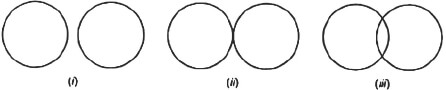# AP Board Class 9 Maths Chapter 12 Circles

In earlier classes, students will have already learnt how to draw a circle with the help of a compass. However, in AP Board Class 9 Maths Chapter 12, students will study more about the Circles and understand the related terms associated with it. They will also go through some important properties of the Circle. Additionally, key topics discussed in this AP Board Class 9 Maths Chapter 12 Circle include:

• Angle subtended by a chord at a point on the circle.
• Perpendicular from the centre to the chord.
• Angle subtended by an arc of a circle.

## What is a Circle?

In plain terms, a circle is said to be a closed curved shape. However, if we read the technical definition, a circle is basically a figure consisting of different points in a plane, which are at an equal distance from a fixed point in the same plane.

Circles having the same radii are called congruent circles, whereas circles with the same centre and different radii are known as concentric circles.

### Some Related Terms

The radius of a circle is usually the fixed distance from the centre. It is also a line segment that connects the centre of a circle to any other point on the same circle.

Diameter

Diameter is nothing but the longest of chords or the line that passes through the centre of a circle.

Arc

An arc is a part between any two points on the circle.

Chord

A chord of a circle is basically a line segment joining any points in the circle.

Sector

A sector is the area of a disk enclosed by two radii and an arc. The circle is usually divided into two specific regions called minor (smaller area ) and major (region having greater area) sector.

Segment

An area enclosed by a chord and an arc is known as a segment.

Students can go through some of the chapter questions along with their solutions below;

Question 1: Fill in the blanks:

(i) The centre of a circle is found in________of the circle.

(ii) The longest chord is a________of the circle.

(iii) An arc is a________when its ends are same as the ends of a diameter.

(iv) A circle’s segment is the area between the arc and________ of the circle.

(v) A circle usually divides the plane, on which it lies, in________parts.

(vi) Circles having a common centre are called_______

Solution: (i) interior (ii) diameter (iii) semi-circle  (iv) the chord (v) three (vi) concentric circles

Question 2: Draw different pairs of circles and determine the points of each pair. Give the maximum number of common points.

Sol: The different pairs of circles need to be drawn as shown below:We then have,

 In figure Maximum Number Of Common Points i Zero ii One iii Two

All in all, two circles will mostly have two points in common.

Keep visiting BYJU’s to know more about Andhra Pradesh Board and access various study materials, including syllabus, exam timetable and more.